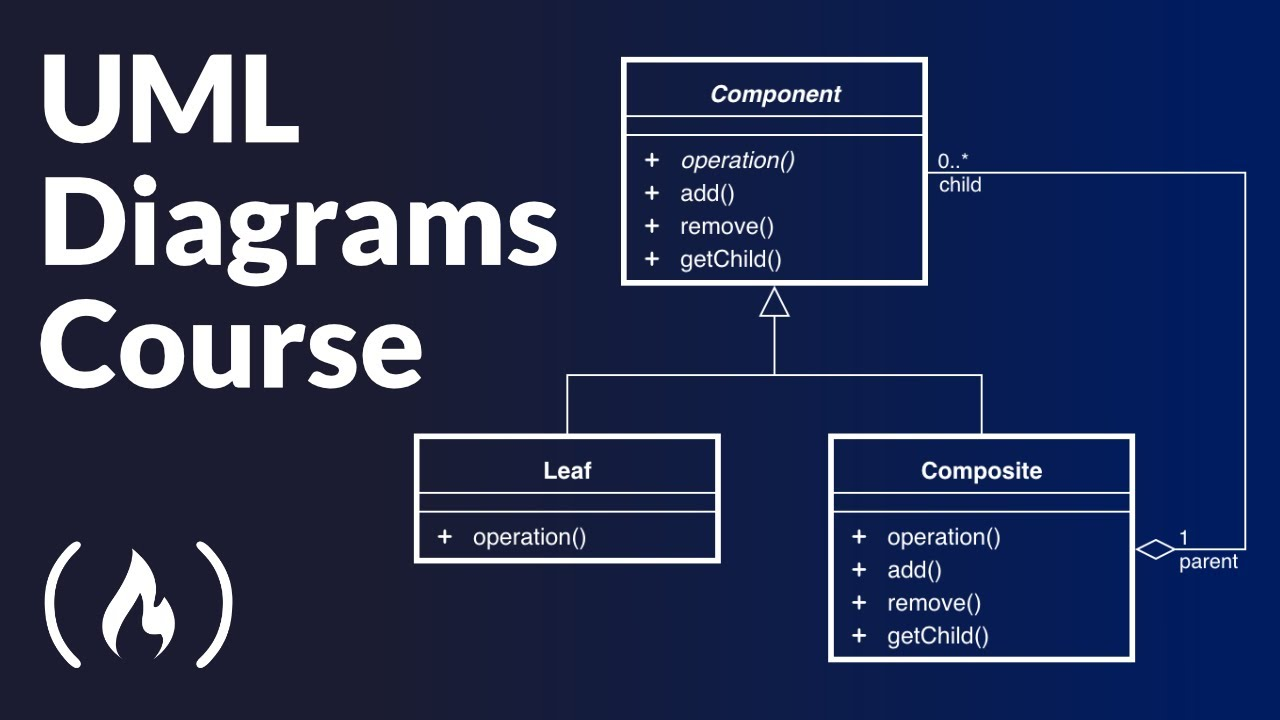# UML Diagrams Full Course (Unified Modeling Language)Learn about how to use UML diagrams to visualize the design of databases or systems. You will learn the most widely used Unified Modeling Language diagrams, their basic notation, and applications. UML diagrams are frequently used in software development.

Learn about how to use UML diagrams to visualize the design of databases or systems. You will learn the most widely used Unified Modeling Language diagrams, their basic notation, and applications. UML diagrams are frequently used in software development.

⭐️ Course Contents ⭐️
⌨️ (0:00:00) Course Introduction
⌨️ (0:02:50) Overview of the main Diagrams in UML 2.0
⌨️ (0:09:39) Class Diagram
⌨️ (0:17:43) Component Diagram
⌨️ (0:25:27) Deployment Diagram
⌨️ (0:31:49) Object Diagram
⌨️ (0:37:41) Package Diagram
⌨️ (0:45:07) Composite Structure Diagram
⌨️ (0:51:32) Profile Diagram
⌨️ (0:57:09) Use Case Diagram
⌨️ (1:04:29) Activity Diagram
⌨️ (1:10:08) State Machine Diagram
⌨️ (1:17:17) Sequence Diagram
⌨️ (1:26:12) Communications Diagram
⌨️ (1:33:57) Interaction Overview Diagram
⌨️ (1:37:11) Timing Diagram